# Calculated Industries Ultra Measure Master Calculator 8025

(No reviews yet)
SKU:
CAL8025
MPN:
8025
•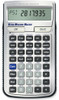•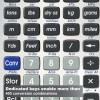•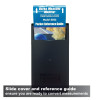•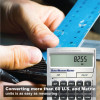•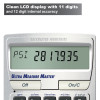•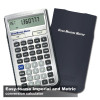•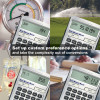•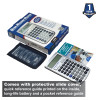\$45.99

## Professional Grade U. S. Standard To Metric Conversion Calculator

The Ultra Measure Master is a complete and easy-to-use U.S. Standard to Metric conversion calculator for engineers, architects, construction pros, scientists and students. Convert more than 60 U.S and Metric (si) units - over 400 conversion combinations with a touch of a few buttons. No need for complex scientific calculators or memorizing conversion formulas.

Dimensional Math and Conversions

• More than 60 standard and metric units built-in for fast, reliable conversions on the job or in the office
• Over 400 conversion combinations
• Works in and convert between feet, inches, fractions, yards, meters, centimeters and millimeters - including square and cubic formats

Dedicated Functions

• Linear: feet-inch-fractions or yards, miles, decimal feet/inch fractions, millimeters, centimeters, meters and kilometers
• Area: feet-inch-fraction, yards, acres, square-miles, millimeters, centimeters and meters, hectares, square-kilometers
• Volume: cubic feet, inch, yards, acre-feet, board feet, millimeters, centimeters, meters, kilometers, miles, gallons, liters and fluid ounces
• Weight: pounds, dry ounces, tons, metric tons, grams and kilograms
• Weight per Volume: tons per cubic yard, pounds per cubic yard, and kilograms per cubic meter
• Linear Velocity: inches and feet per second, feet per minute, miles per hour, minute and hour, millimeters and meters per second /minute and kilometers per hour
• Flow Rate: ounces per second, gallons per minute, milliliters and liters per second
• Pressure: pounds per square inch (PSI), pounds per square foot (PSF), kilo-Pascal, mega-Pascal and Bars
• Bending Moment: inch-pounds, foot-pounds and Newton Meters
• Temperature: Fahrenheit (°F) and Centigrade (°C).

• User Preferences - set custom options: Fractions, Area, Volume
• Cost per Unit: Solve unit cost and pricing
• Backspace Key: Easily correct entry errors
• "Paperless" Tape: Allows review of the last 20 entries; double-check totals.
• Standard Calculations: works as a math calculator with %, +, -, ×, ÷, +/-, π, 1/x, x2, and √

Includes

• Protective Slide Cover
• One 3-Volt CR-2016 Lithium Battery

Support Materials

View AllClose

## Warranty Information

Includes one-year warranty from Calculated Industries
View AllClose